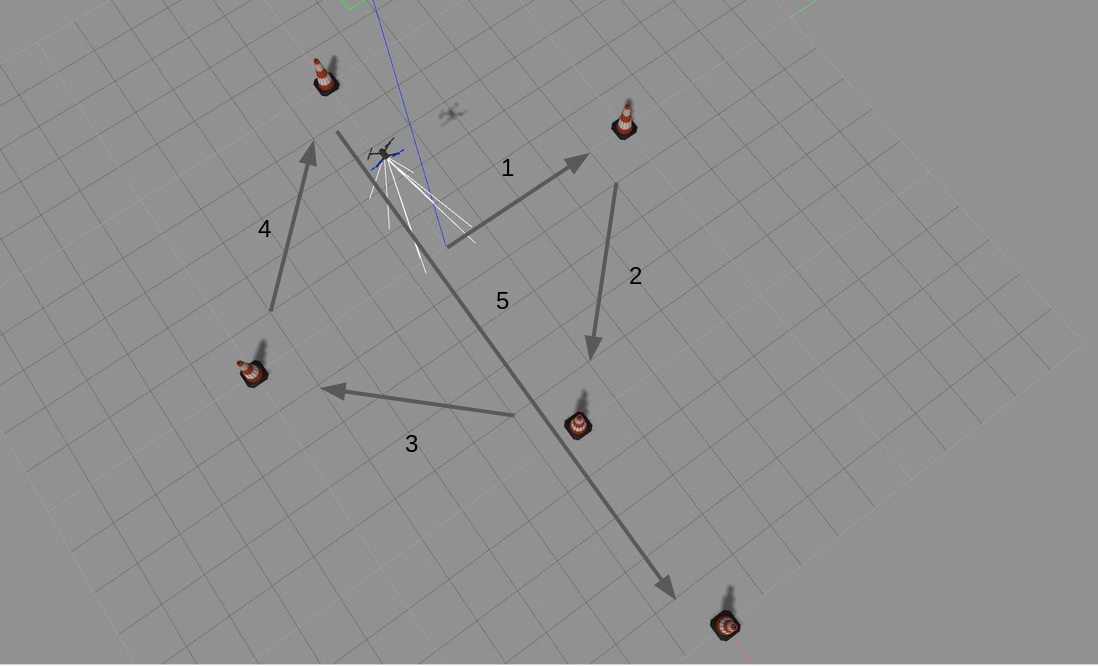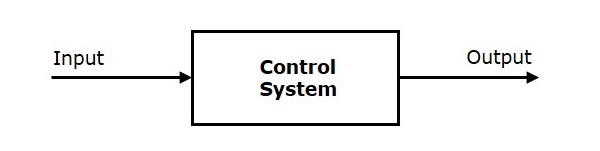# Position Control

The goal of this exercise is to implement a local navigation algorithm through the use of a PID controller.

For this exercise, a world has been designed in gazebo that contains the Iris quadrotor and 5 beacons arranged in a cross. The task is to program the drone to follow the route as given in the picture below.Gallery.

## Installation

Install the General Infrastructure of the JdeRobot Robotics Academy.

As this is a drones exercise, you will need to additionally install the jderobot-assets, dronewrapper and rqt_drone_teleop packages. These can be installed as

sudo apt-get install ros-melodic-drone-wrapper ros-melodic-rqt-drone-teleop ros-melodic-jderobot-assets


There is an additional dependency on MAVROS and PX4 that you can fulfill following the Drones installation instructions.

## How can I run the exercise?

To launch the exercise, simply use the following command from this directory:

roslaunch position_control.launch


## How should I solve the exercise?

To solve the exercise, you must edit the my_solution.py file and insert the control logic into it.

### Where to insert the code

Your code has to be entered in the execute function between the Insert your code here comments.

my_solution.py

def execute(event):
global drone
img_frontal = drone.get_frontal_image()
img_ventral = drone.get_ventral_image()
# Both the above images are cv2 images
################# Insert your code here #################################

set_image_filtered(img_frontal)
set_image_threshed(img_ventral)

#########################################################################


You must assume your drone has already took off from the central green pad and its already airbone (i.e. you already pressed the Takeoff button). Then your drone has to navigate autonomously reaching each beacon.

To remember: At the moment, each time you update your code you must to run again the launch file in order to insert the updated code in the drone teleoperator GUI

## API

### Sensors and drone state

• drone.get_position() - Returns the actual position of the drone as a numpy array [x, y, z], in m.
• drone.get_velocity() - Returns the actual velocities of the drone as a numpy array [vx, vy, vz], in m/s
• drone.get_yaw_rate() - Returns the actual yaw rate of the drone, in rad/s.
• drone.get_orientation() - Returns the actual roll, pitch and yaw of the drone as a numpy array [roll, pitch, yaw], in rad.
• drone.get_roll() - Returns the roll angle of the drone, in rad
• drone.get_pitch() - Returns the pitch angle of the drone, in rad.
• drone.get_yaw() - Returns the yaw angle of the drone, in rad.
• drone.get_landed_state() - Returns 1 if the drone is on the ground (landed), 2 if the drone is in the air and 4 if the drone is landing. 0 could be also returned if the drone landed state is unknown.

### Actuators and drone control

The three following drone control functions are non-blocking, i.e. each time you send a new command to the aircraft it immediately discards the previous control command.

#### 1. Position control

• drone.set_cmd_pos(x, y, z, az) - Commands the position (x,y,z) of the drone, in m and the yaw angle (az) (in rad) taking as reference the first takeoff point (map frame)

#### 2. Velocity control

• drone.set_cmd_vel(vx, vy, vz, az) - Commands the linear velocity of the drone in the x, y and z directions (in m/s) and the yaw rate (az) (rad/s) in its body fixed frame

#### 3. Mixed control

• drone.set_cmd_mix(vx, vy, z, az) - Commands the linear velocity of the drone in the x, y directions (in m/s), the height (z) related to the takeoff point and the yaw rate (az) (in rad/s)

### Drone takeoff and land

Besides using the buttons at the drone teleoperator GUI, taking off and landing can also be controlled from the following commands in your code:

• drone.takeoff(height) - Takeoff at the current location, to the given height (in m)
• drone.land() - Land at the current location.

### Drone cameras

• drone.get_frontal_image() - Returns the latest image from the frontal camera as a OpenCV cv2_image
• drone.get_ventral_image() - Returns the latest image from the ventral camera as a OpenCV cv2_image
• set_image_filtered(cv2_image) - Shows a filtered image of the camera images in the GUI
• set_image_threshed(cv2_image) - Shows a thresholded image in the GUI

### Beacons

• init_beacons() - Initializes the beacons
• get_next_beacon() - Returns the next beacon to be visited
• beacon.get_pose() - Returns pose of the beacon
• beacon.get_id() - Returns id of the beacon
• beacon.is_reached() - Returns reached of the beacon
• beacon.set_reached(value) - Sets reached parameter of a beacon
• beacon.is_active() - Returns active of the beacon
• beacon.set_active(value) - Sets active parameter of a beacon

## Theory

PID Control is the main fundamental behind this exercise. To understand PID Control, let us first understand what is Control in general.

### Control System

A system of devices or set of devices, that manages, commands, directs or regulates the behavior of other devices or systems to achieve the desired results. Simply speaking, a system which controls other systems. Control Systems help a robot to execute a set of commands precisely, in the presence of unforeseen errors.

### Types of Control System

#### Open Loop Control System

A control system in which the control action is completely independent of the output of the system. A manual control system is on Open Loop System.

#### Closed Loop Control System

A control system in which the output has an effect on the input quantity in such a manner that the input will adjust itself based on the output generated. An open loop system can be converted to a closed one by providing feedback.

### PID Control

A control loop mechanism employing feedback. A PID Controller continuously calculates an error value as the difference between desired output and the current output and applies a correction based on proportional, integral and derivative terms(denoted by P, I, D respectively).

• Proportional

Proportional Controller gives an output which is proportional to the current error. The error is multiplied with a proportionality constant to get the output. And hence, is 0 if the error is 0.

• Integral

Integral Controller provides a necessary action to eliminate the offset error which is accumulated by the P Controller.It integrates the error over a period of time until the error value reaches to zero.

• Derivative

Derivative Controller gives an output depending upon the rate of change or error with respect to time. It gives the kick start for the output thereby increasing system response.Control Systems and PID

### Tuning Methods

In order for the PID equation to work, we need to determine the constants of the equation. There are 3 constants called the gains of the equation. We have 2 main tuning methods for this.

• Trial and Error

It is a simple method of PID controller tuning. While system or controller is working, we can tune the controller. In this method, first we have to set Ki and Kd values to zero and increase proportional term (Kp) until system reaches to oscillating behavior. Once it is oscillating, adjust Ki (Integral term) so that oscillations stops and finally adjust D to get fast response.

• Zeigler Nichols method

Zeigler-Nichols proposed closed loop methods for tuning the PID controller. Those are continuous cycling method and damped oscillation method. Procedures for both methods are same but oscillation behavior is different. In this, first we have to set the p-controller constant, Kp to a particular value while Ki and Kd values are zero. Proportional gain is increased till system oscillates at constant amplitude.

## Hints

Simple hints provided to help you solve the position_control exercise. Please note that the full solution has not been provided.

### How do I know the position of each beacon?

You can use the beacon API proposed in the exercise.

### Directional control. How should drone yaw be handled?

If you don’t take care of the drone yaw angle or yaw_rate in your code (keeping them always equal to zero), you will fly in what’s generally called Heads Free Mode. The drone will always face towards its initial orientation, and it will fly sideways or even backwards when commanded towards a target destination. Multi-rotors can easily do that, but what’s not the best way of flying a drone.

In this exercise, your drone should follow the path similarly to how a fixed-wing aircraft would do, namely nose forward. Then, you’ll have to implement by yourself some kind of directional control, to rotate the nose of your drone left or right using yaw angle, or yaw_rate.

However, you can first solve the exercise ignoring the yaw angle (heads free) and the improve it using a nose forward mode.

This might help, if you know your current position and your target one, you can easily compute the direction (yaw angle) the drone must be turned to by applying some elementary geometry. Probably both math.sqrt() and math.atan2() Python functions will be very useful for you here.

### Coding the Controller

The Controller can be designed in various configurations. 3 configurations have been described in detail below:

• P Controller The simplest way to do the assignment is using the P Controller. Just find the error which is the difference between our Set Point (the point where our drone should be heading) and the Current Output (where the drone is actually heading). Keep adjusting the value of the constant, till we get a value where there occurs no unstable oscillations and no slow response.

• PD Controller This is an interesting way to see the effect of Derivative on the Control. For this, we need to calculate the derivative of the output we are receiving. Since, we are dealing with discrete outputs in our case, we simply calculate the difference between our previous error and the present error, then adjust the proportional constant. Adjust this value along with the P gain to get a good result.

• PID Controller This is the complete implemented controller. Now, to add the I Controller we need to integrate the output from the point where error was zero, to the present output. While dealing with discrete outputs, we can achieve this using accumulated error. Then, comes the task of adjustment of gain constants till we get our desired result.

### Do I need to know when the drone is in the air?

No, you can solve this exercise without taking care of the land state of the drone. However, it could be a great enhancement to your blocking position control function if you make it only work when the drone is actually flying, not on the ground.